Back
Relative References in Excel
> ... Math > Excel > Relative References in Excel

You can watch the video or just read the text below. Both will give you the same information.

Introduction to relative references in Excel

### Referencing Cells

To reference another cell, type the = symbol and then the name of the cell you want to reference.

Example:

We want to reference the value in cell A1 in cell A3. The number 2 is typed into A1.

Next, select the cell A3 and type “=A1” (without the quotation marks).

You can either type =A1 or you can type the = symbol and then click on the cell A1. This will also automatically enter the name of the cell A1 into the new cell.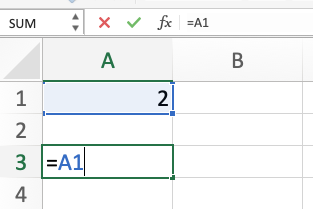Press Enter.

The value of 2 from cell A1 appears in cell A3. Notice that the formula bar still shows “=A1” as the contents of cell A3, but the value from A1 is what we see in the cell.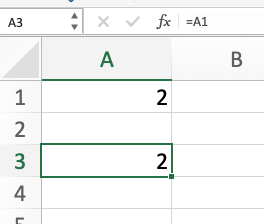The benefit of doing this is that if we change the value in cell A1, the value in our referenced cell automatically changes too.

Example: Change the value in A1 to 9 and type enter. The value in A3 automatically changes to 9 as well.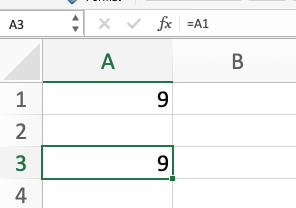### Using Cell References in an Equation

Excel can take the values from different cells and plug them into all kinds of equations and formulas. It does this by referencing cells. You can reference more than one cell at a time as part of an equation.

Example: Add the values of two cells together.

Cell A1 contains the number 9.

Cell B1 contains the number 2.

In cell C1 we will add these two values together by typing “=A1+B1” (without quotation marks).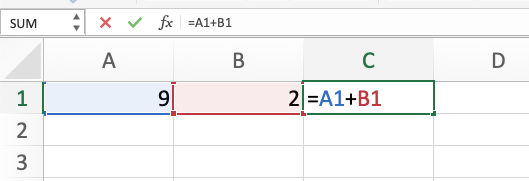Press Enter.

The value 11 will appear in cell C1.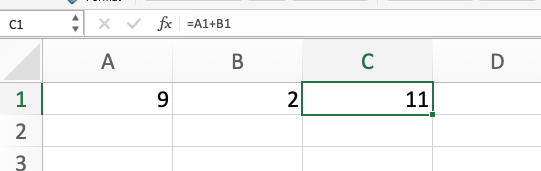If we change any of the values in cells A1 or B1, the value in C1 will automatically update.

Example: If we change the value of B1 to 9, then C1 automatically updates to 18 which is 9+9.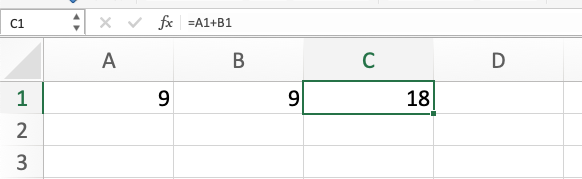Referencing cells can be used with all kinds of formulas and equations in Excel. See the lesson on multiplication in Excel for another example of referencing cells.

### Relative References in Excel are Variables

Here is an explanation of how Excel uses references as variables.

Relative References in Excel

## Need More Help?

1. Study other Math Lessons in the Resource Center.
2. Visit the Online Tutoring Resources in the Resource Center.